Next: 6.2.5 The New Method Up: 6.2 Circuit Equation Damping Previous: 6.2.3 Global Damping Strategy

## 6.2.4 Local Limiting

In conventional circuit simulation it is common practice to limit the contact voltages before evaluating the compact model. This is illustrated for a simple diode model represented by the equation

 I = IS .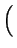exp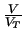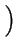- 1(6.3)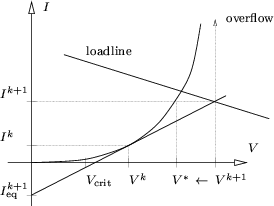with IS being the saturation current and VT = kB . T/q the thermal voltage. At the Newton iteration step k equation (6.3) is linearized around the current solution point Vk. The solution of the linearized system is found as Vk + 1. However, directly using Vk + 1 (vertical projection) can easily lead to overflow of the exponential function in (6.3) as shown in Fig. 6.1. To overcome this problem, horizontal projection has been introduced. The appropriate expressions are easily derived using Fig. 6.1. The current change evaluates to

 Ik + 1 - Ik =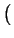Vk + 1 - Vk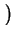.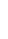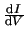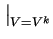. (6.4)

Inserting (6.3) gives
 exp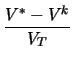= 1 +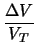(6.5)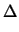V = Vk + 1 - Vk (6.6)

and finally

 V* = Vk + VT . ln1 +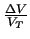. (6.7)

However, horizontal projection is only useful for Vk + 1 > Vcrit. Below Vcrit vertical projection obviously delivers better results. Equation (6.7) can be generalized to arbitrary non-linearities 

 V* = Vk +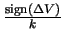. ln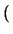1 + k . |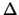V|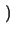(6.8)

with k being a new scaling factor which can be optimized for each single device in the course of iteration progress. A larger k gives stronger damping and hence increases reliability of the algorithm for the price of longer simulation times. It must be pointed out that this mixed method of updating is not amenable to an easy description in terms of matrices, Jacobians, etc., and hence convergence theorems for this method are impossible to formulate. However, actual experience with this procedure in handling all types of circuits during the last decades has proven its effectiveness. As the voltages are damped in an individual manner for each device this damping algorithm will be termed local in the following. When investigating (6.3) one notices that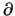I/V can become very small for V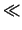0 which could result in a singular matrix. To prevent this occurrence a small leakage conductance Gmin of typically 10-9- 10-12 S is placed in shunt with each junction. Alternatively Gmin can be connected between each device node and ground which is similar from an electrical point of view but improves diagonal dominance compared to a shunt conductance. In addition, the exponential characteristic is replaced by a linear characteristic for bias voltages smaller than a few VT. Although the above mentioned precautions are guided by numerical reasoning it should be noticed that they do not have any negative impact on the value of the solution as they might as well be justified on physical grounds.Next: 6.2.5 The New Method Up: 6.2 Circuit Equation Damping Previous: 6.2.3 Global Damping Strategy
Tibor Grasser
1999-05-31